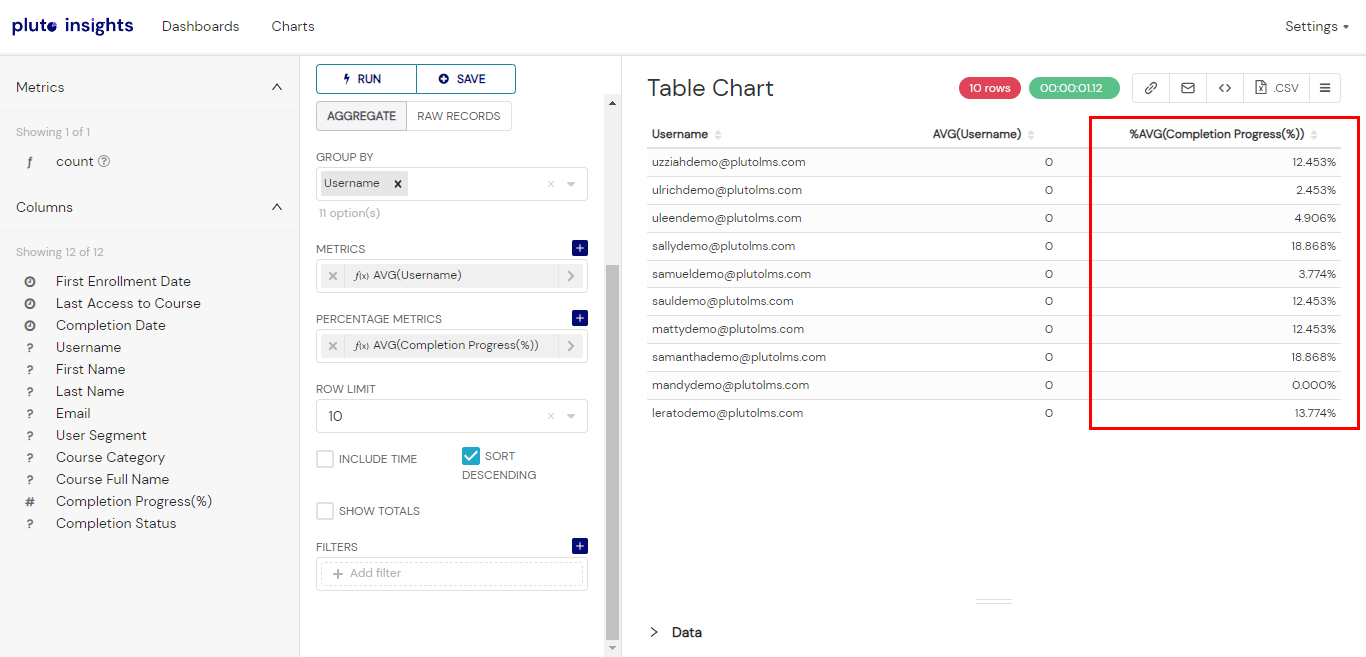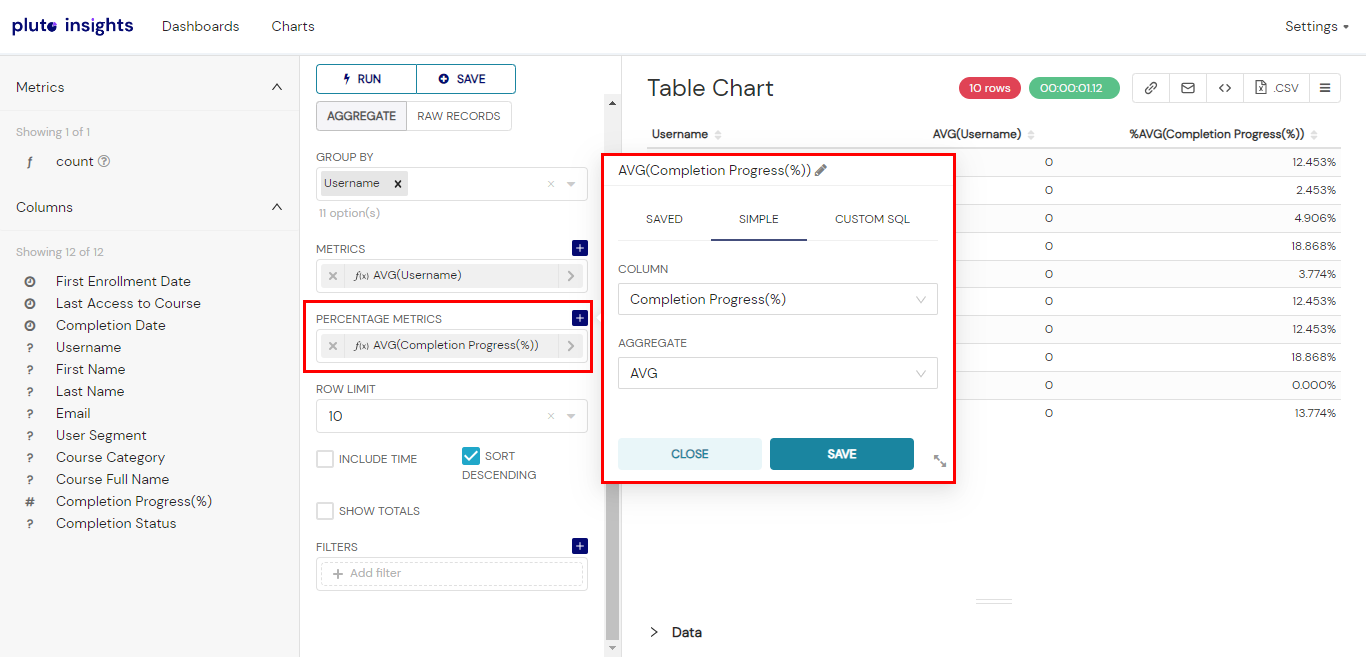# Percentage Metrics

### OVERVIEW

Percentage metrics in Pluto Insights are metrics that represent a value as a percentage of a total or a subset of the data. They are useful for comparing proportions or relative contributions of different categories within your dataset.### HOW TO CALCULATE PERCENTAGE METRICS

To calculate percentage metrics, you typically combine an aggregate function with a specific column or set of columns that define the denominator or subset of the data.

Here are a couple of examples:

> Percentage of Total: This metric calculates the percentage of a value in relation to the total. For instance, you might want to visualize the percentage of total enrollments contributed by each course category. To do this, select the "Sum" aggregate function for the enrollments column and divide it by the total enrollments across all categories. The resulting percentage metric will show the relative contribution of each course category to the overall enrollments.

> Percentage of Subgroup: This metric calculates the percentage of a value within a specific subgroup or category. For example, you may want to compare the percentage of learners who completed courses within different companies. To do this, use the "Count" aggregate function to count the number of learners within each company and divide it by the total number of learners. The resulting percentage metric will show the proportion of learners in each company.Percentage metrics help you gain insights into the relative distribution or contribution of different categories or subsets of your data. By visualizing these percentages in charts, you can effectively compare and analyze proportions across various dimensions.

• # Related Articles

• ## Metrics

Overview In Pluto Insights, "metrics" refer to the quantitative measurements or calculations that you want to display or analyze in your charts. Metrics provide numerical values that represent specific aspects of your data. When creating a chart, you ...
• ## How to create a Pie chart

OVERVIEW A pie chart is a circular graphical representation used to display data as a proportional distribution of categories. The chart resembles a pie, with each category represented as a slice, where the size of each slice corresponds to the ...
• ## How to create a Bar chart

Overview A Bar Chart compares metrics from different categories using bars. Bar lengths are used to indicate the magnitude of each value and colour is used to differentiate groups. Creating a Bar Chart Step 1: Select the "Charts" tab in the top bar, ...
• ## How to create a Big Number chart

OVERVIEW Showcases a single metric front-and-center. Big number is best used to call attention to a KPI or the one thing you want your audience to focus on. The intention of a big number chart is to visually highlight and draw attention to important ...
• ## Time Column

A time column is a specific field in your dataset that holds time information. This could be a timestamp or a date, and it is usually used in data visualizations to track changes over a period of time. In Pluto Insights, the time column can be used ...